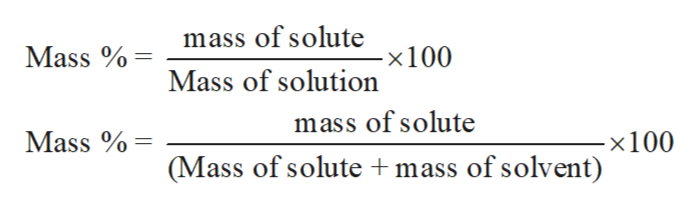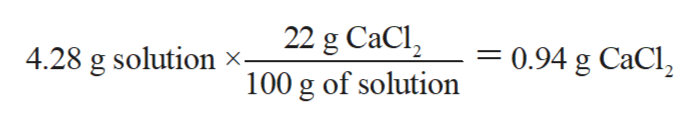# Calculate the milligrams of chlorine (35.453 g/mol) in a 4.28 g solution of 22.2 wt% CaCl2 (110.98 g/mol).

Question
487 views

Calculate the milligrams of chlorine (35.453 g/mol) in a 4.28 g solution of 22.2 wt% CaCl2 (110.98 g/mol).

check_circle

Step 1

The concentration of any substance can be expressed in different ways such as molality, molarity, normality etc. Some other ways are ppm, ppb, mole fraction and mass percentage.

Step 2

Mass percent or weight percent of a solution is the mass of the solute by the mass of the solution multiply by 100. The mathematical expression for molality can be written as:help_outlineImage Transcriptionclosemass of solute Mass x 100 Mass of solution mass of solute -x 100 (Mass of solute mass of solvent) Mass fullscreen
Step 3

The mass percentage as 22% of CaCl2 depicted as 22 g of CaCl2 is dissolved in 100 g of...help_outlineImage Transcriptionclose22 g CaCl = 0.94 g CaCl 4.28 g solution x- 100 g of solution fullscreen

### Want to see the full answer?

See Solution

#### Want to see this answer and more?

Solutions are written by subject experts who are available 24/7. Questions are typically answered within 1 hour.*

See Solution
*Response times may vary by subject and question.
Tagged in

### Chemistry